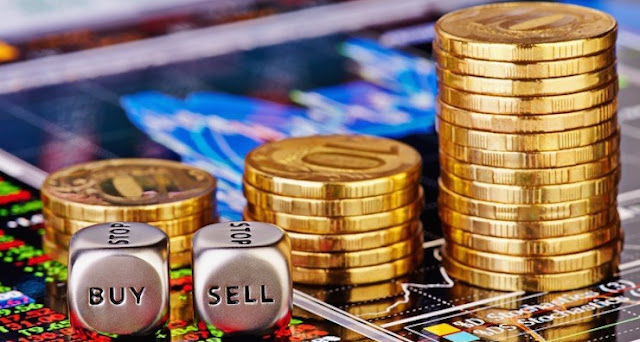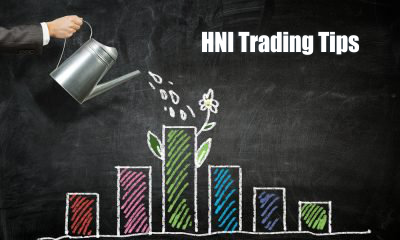## Posts

### How to measure risk in the commodity market?The risk is defined by the bullion tips providers as the deviation of actual returns from expected return. Hence measurement of risk needs data for actual as well as expected returns. Thus, while evaluating the risk of an investment two types of information are needed: 1. Information on the future values of return from that investment. 2. The likelihood of occurrence, or probability estimate for each return value.   However, in real life, we may not be able to estimate future returns, or even correctly decide the range of values that returns could take. Nor can we assign accurate probabilities to each one of these values. Investors only have a single series of past observations, from which both actual and expected returns have to be extracted. Since most investors tend to form expectations on the basis of historical performance of an asset, it is a common practice to represent expected returns by an average return. For example, suppose the annual return from e

### What Do You Mean By Exchange Rate Risk?Exchange rate risk is incurred due to changes in the exchange rate of domestic currency relative to a foreign currency. When a domestic investor invests in foreign assets, or a foreign investor invests in domestic assets, the investment of HNI traders is subject to exchange rate risk.  It must be noted that: If domestic currency depreciates (falls in value) against foreign currency, the value of foreign asset goes up in terms of domestic currency and the value of domestic assets in terms of foreign currency goes down. If domestic currency appreciates (increase in value) against foreign currency, the value of foreign asset goes down in terms of domestic currency and the value of the domestic assets in terms of foreign currency goes up. Consider this example. An NRI based in the US invests \$1000 in a  bank deposit in India at10% for 1 year when the exchange rate is Rs. 60 per US\$. After one year, the rupee depreciates and the exchange rate is Rs. 67 per US\$. What is the risk t